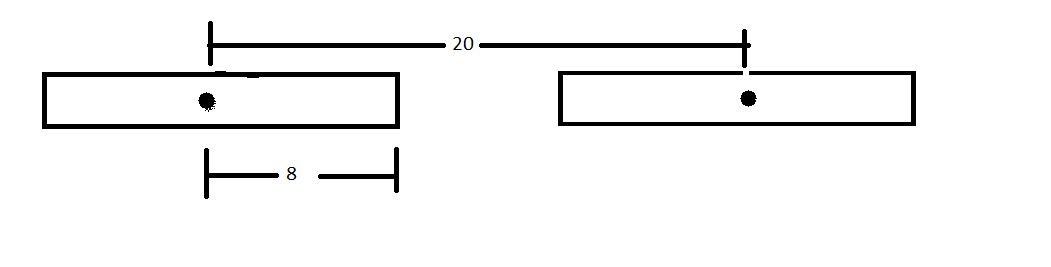# Simple isn't it? #3Consider two identical sticks of length 16 m, each carrying charge 40 C. The distance between their centers is 20 m. Calculate the force of repulsion on each stick due to the other stick.

Details

• Take $\displaystyle\frac{1}{4\pi \varepsilon_0 } =9\times 10^9\ \text{Nm}^2/\text{C}^2$.
×